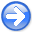Time-shift imaging condition in seismic migrationNext: Angle transformation in wave-equation Up: Imaging condition in wave-equation Previous: Time-shift imaging condition

## Space-shift and time-shift imaging condition

To be even more general, we can formulate an imaging condition involving both space-shift and time-shift, followed by image extraction at zero time:(9)(10)

However, the cost involved in this transformation is large, so this general form does not have immediate practical value. Imaging conditions described by equations  (3)-(4) and (6)-(7) are special cases of equations (9)-(10) forand, respectively.

2007-04-08